# Brooks-Jewett theorem

(diff) ← Older revision | Latest revision (diff) | Newer revision → (diff)

Letbe a topological group. A set function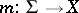is exhaustive (also called strongly bounded) iffor each sequence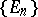of pairwise disjoint sets from the-algebra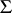(cf. also Measure). A sequenceof set functions,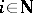, is uniformly exhaustive ifuniformly infor each sequenceof pairwise disjoint sets from the-algebra.

Being a generalization of the Nikodým convergence theorem, the Brooks–Jewett theorem [a1] says that for a pointwise-convergent sequenceof finitely additive scalar and exhaustive set functions (strongly additive) defined on a-algebra, i.e. such that,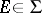:

i)is an additive and exhaustive set function;

ii)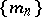is uniformly exhaustive.

There is a generalization of the Brooks–Jewett theorem for-triangular set functions defined on algebras with some weak-conditions (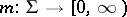is said to be-triangular forif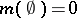and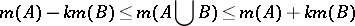whenever,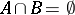). The following definitions are often used [a2], [a6], [a5]:

SCP) An algebrahas the sequential completeness property if each disjoint sequencefromhas a subsequence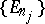whose union is in.

SIP) An algebrahas the subsequentional interpolation property if for each subsequence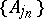of each disjoint sequence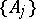fromthere are a subsequence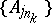and a set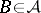such that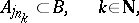and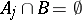for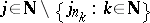.

According to [a5]: Letsatisfy SIP) and let,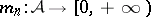,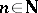, be a sequence of-triangular exhaustive set functions. If the limit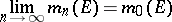exists for each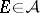andis exhaustive, thenis uniformly exhaustive and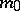is-triangular.

There are further generalizations of the Brooks–Jewett theorem, with respect to: the domain of the set functions (orthomodular lattices,-posets); properties of the set functions; and the range (topological groups, uniform semi-groups, uniform spaces), [a2], [a4], [a5].

It is known that for additive set functions the Brooks–Jewett theorem is equivalent with the Nikodým convergence theorem, and even more with the Vitali–Hahn–Saks theorem [a3].